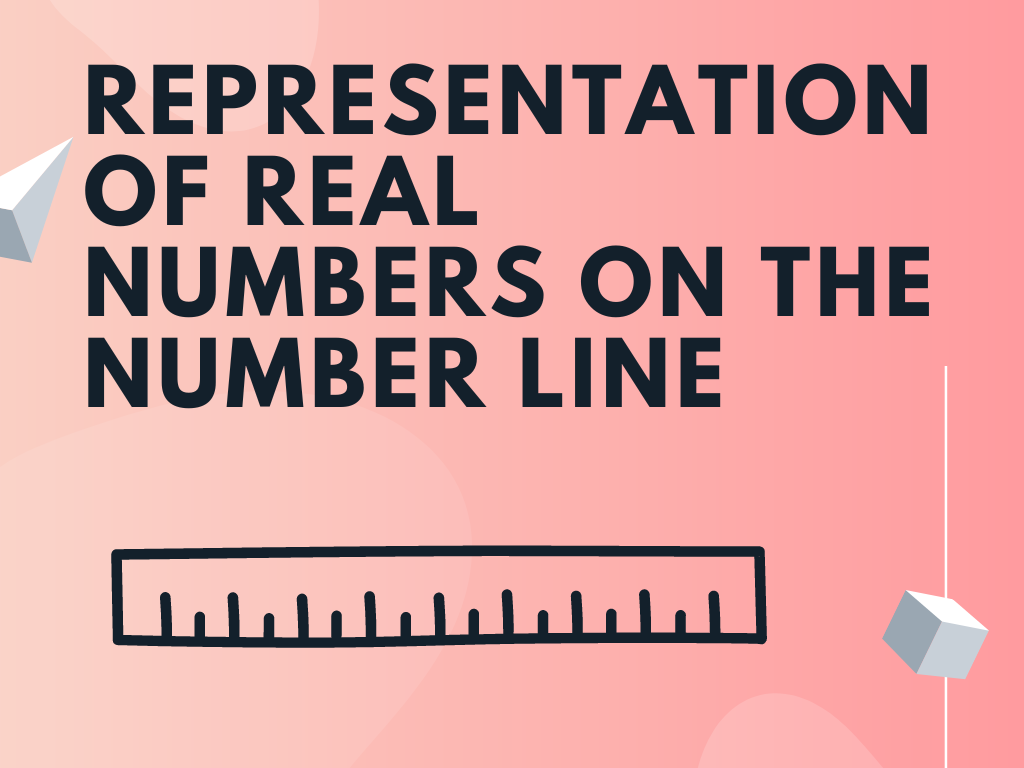# class 9 real numbers## Operations on Real Numbers Class 9th

In this section, we shall study different mathematical operations on real numbers such as addition, subtraction, multiplication, and division. We know that rational numbers and irrational numbers are real numbers so we’ll apply operations on them. For Rational Numbers As we have learnt in previous classes, rational numbers satisfy the commutative, associative, and distributive properties …## Representation of Real Numbers on the Number Line Class 9th

As per the knowledge of the previous classes, we are familiar with the representation of different types of numbers on the number line like the representation of natural numbers, whole numbers, integers, and rational numbers. In this section, we’ll learn the representation of real numbers on the number line including irrational numbers also. We’ll represent …

Scroll to Top